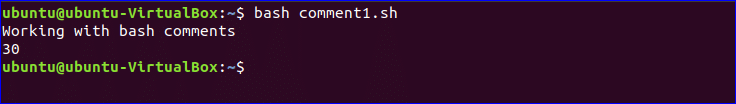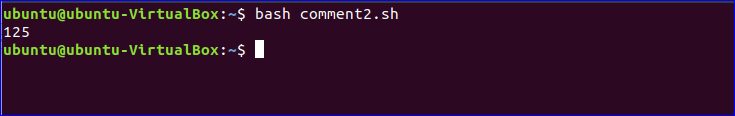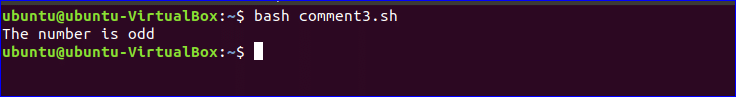# How to use single and multiple line comments in BASH

Using comments in any script or code is very important to make the script more readable. Comments work as a documentation for the script. The reader can easily understand each step of the script if it is properly commented by the author. Comments are ignored when the script executes. The single line can be commented very easily in the bash script. But there are multiple ways to comment multiple lines in the bash script. How you can use single and multiple lines comments in bash scripts is shown in this tutorial.

## Single line comment:

You can explain the function of each line of the script by adding single line comment on the above or side of the line. ‘#’ symbol is used to comment single line in the bash script. The following example shows the use of single line comment.

### Example-1: Single line comment

#!/bin/bash
#Print a simple text
#Add 10 with 20 and store the value in n
((n=10+20))
#Print the value of n
echo \$n

Output:

Three single line comments are used in the above script and those lines are ignored in the output.There is no direct option to comment multiple lines in the bash script. You can use other features of bash to comment multiple lines in a script. One option is using ‘here document’ and another option is using ‘:’. The uses of both options are shown in the following examples.

### Example-2: Multiple line Comment using here document

Here, LongComment is used as here document delimiter to add the multiline comment.

#!/bin/bash
<<LongComment
This script is used to
Calculate the cube of
a number with value 5
LongComment

#Set the value of n
n=5
#Calculate 5 to the power 3
((result=\$n*\$n*\$n))
#Print the area
echo \$result

Output:

All comments are ignored in the output.### Example-3: Multiline comment using ‘:’ command

Write the multiline comment using single quote after ‘:’.

#!/bin/bash
#Initialize the variable n with a number
n=15
: '
The following script will check the number is
Even or odd by dividing the number by 2 and checking the remainder value
'

if (( \$n % 2 == 0 ))
then
echo "The number is even"
else

echo "The number is odd"
fi

Output:

All comments are ignored in the output.